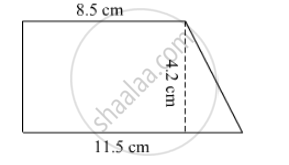SSC (English Medium) Class 8Maharashtra State Board
Share

# Length of the Two Parallel Sides of a Trapezium Are 8.5 Cm and 11.5 Cm Respectively and Its Height is 4.2 Cm, Find Its Area. - SSC (English Medium) Class 8 - Mathematics

ConceptArea of a Trapezium

#### Question

Length of the two parallel sides of a trapezium are 8.5 cm and 11.5 cm respectively and its height is 4.2 cm, find its area.

#### SolutionArea of trapezium = 1/2 (sum of parallel sides) x height

= 1/2 x (8.5 + 11.5) x 4.2

= 1/2 x 20 x 4.2

= 42 cm²

Is there an error in this question or solution?

#### APPEARS IN

Balbharati Solution for Balbharati Class 8 Mathematics (2019 to Current)
Chapter 15: Area
Practice Set 15.3 | Q: 2 | Page no. 99
Solution Length of the Two Parallel Sides of a Trapezium Are 8.5 Cm and 11.5 Cm Respectively and Its Height is 4.2 Cm, Find Its Area. Concept: Area of a Trapezium.
S